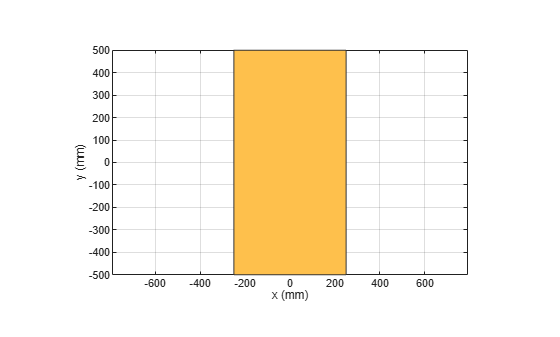# scale

Change the size of the shape by a fixed amount

## Syntax

``c = scale(shape,scaling)``

## Description

example

````c = scale(shape,scaling)` scales the shape by a constant factor```

## Examples

collapse all

Create a rectangular shape.

```r = antenna.Rectangle; show(r) axis equal```Shrink the rectangle by 50%.

`scale(r,0.5);`## Input Arguments

collapse all

Shape created using custom elements and shape objects of Antenna Toolbox, specified as an object.

Example: `c = scale(rectangle1,0.5)` where rectangle1 is created using `antenna.Rectangle` object.

Constant factor to change shape size, specified as a scalar.

Data Types: `double`